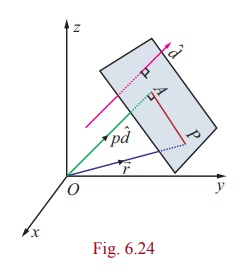Home | | Maths 12th Std | Equation of a plane when a normal to the plane

# Equation of a plane when a normal to the plane

Equation of a plane when a normal to the plane and the distance of the plane from the origin are given (a) Vector equation of a plane in normal form (b) Cartesian equation of a plane in normal form

Equation of a plane when a normal to the plane and the distance of the plane from the origin are given

## (a) Vector equation of a plane in normal form

Theorem 6.15

The equation of the plane at a distance p from the origin and perpendicular to the unit normal vector dˆ isdˆ = p .

Proof

Consider a plane whose perpendicular distance from the origin is p

Let A be the foot of the perpendicular from to the plane.

Let dˆ be the unit normal vector in the direction of.Then= pdˆ .

Ifis the position vector of an arbitrary point P on the plane,

thenis perpendicular to.The above equation is called the vector equation of the plane in normal form.

## (b) Cartesian equation of a plane in normal form

Let l, m, n be the direction cosines of dˆ. Then we have dˆ = liˆ + mˆj + nkˆ.

Thus, equation (1) becomes. (liˆ + mˆnkˆ) = p

If P is (x,y,z), then= xˆi + yˆj + zˆk

Therefore, (xiˆ + yˆj + zkˆ) (liˆ + mˆj + nkˆ) = p or lx + my + nz = p                     ............(2)

Equation (2) is called the Cartesian equation of the plane in normal form.

Remark

(i) If the plane passes through the origin, then p = 0 . So, the equation of the plane is lx + my + nz = 0.

(ii) Ifis normal vector to the plane, then ˆd =is a unit normal to the plane. So, the vector equation of the plane is= p or.= q , where q = p || . The equation.= q is the vector equation of a plane in standard form.

Note

In the standard form.= q ,need not be a unit normal and q need not be the perpendicular distance.

Tags : Definition, Theorem, Proof , 12th Mathematics : UNIT 6 : Applications of Vector Algebra
Study Material, Lecturing Notes, Assignment, Reference, Wiki description explanation, brief detail
12th Mathematics : UNIT 6 : Applications of Vector Algebra : Equation of a plane when a normal to the plane | Definition, Theorem, Proof